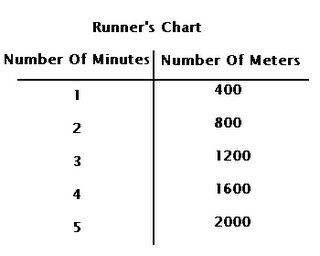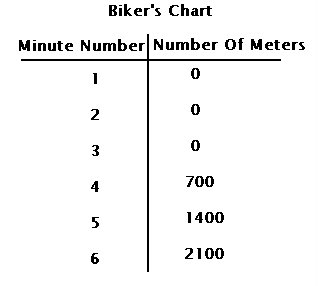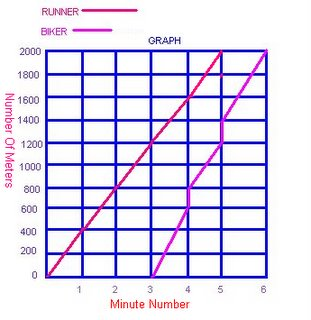## Thursday, June 01, 2006

### Growing Post

Question 1: What is the difference between an algerbraic expression and an equation (Hint: One contains this and the other doesn't)? Give an example of both an expression and an equation.

An Algebraic expression is when you combine numbers, operatives, and/or free variables and bound variables. You get to choose the variable in an algebraic expression. An example: 5A X 6.
In Algebraic equation, the variable is already given. There are two types of equations (from my knowledge), one step; 3E - 6 = 9, and two step; 3N = 1N +10. An example of an equation; 3H - 5 = 10. H = 5.

Question 2: What is a variable and why do we use one in algebra?

Variables are letters that represent the unknown, able to change. Variables are often contrasted with constants, which are known and unchangning.
We use variables because Algebra is a method of finding the unknown.

Question 3: Solve for N

To solve the first question, you need to figure out what a triangle equals on the first scale. First, take away four circles (each square equals two circles), which leaves five circles. Since there were five circles left, the triangle equals five circles.
To solve the second question, you need to figure out the value of the two N's.
First, add the squares and triangles together. The total of the squares and triangles, together, was 22 circles. Then divide the 22 circles by 2. The answer was 11. Each N equals 11 circles.

Question 4: Solve the following equations. Show all of the steps that are needed.

A) 3n+4n+7=2n+12

3N + 4N - 2N = 12 - 7
5N = 5
N=1

B) 8n-(4+9)=11

8N = 13 + 11

13 + 11 = 24

8N = 24

N = 3

C) (8-3)n+7n+8= 4n + 40

5N + 7N + 8= 4N + 40
5N + 7N - 4N = 40 - 8

8N = 32

N = 4

Question 5: For this question you need to create a T-Chart and a graph to plot your data from this question onto.
You are having a race against your friend, except you are on foot and he is on his bike. Your both know that if you are on a bike you will be faster so your friend gives you a head start of 3 minutes. If you can run 400m per minute, and your friend can bike 700m per minute, who will be the first to make it to the
finish line 2000m away?
The runner will finish in five minutes, the bicyclist will finish in six minutes, therefore the runner winning.At 6/01/2006 3:50 PM,Mr. Reece said...

You did a great job with this post Dragon's eye. the only change that I can recommend is that you are not solving for how many circles, as the circles have a value in question 3

Great job

At 6/19/2006 2:32 PM,Mr. Reece said...

Your mark is 29/30 as you did not solve for a value, you solved for circles with question 3, yet each circle has a value.

At 1/17/2018 7:29 PM,learn to fly 2 said...

I’m glad you enjoyed it. Those are great habits! Thank you for sharing.
lennyfacetext.com